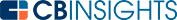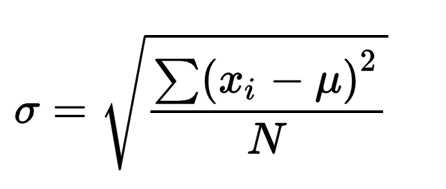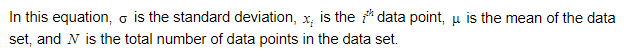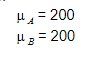# What is the Standard Deviation Formula?

Standard deviation is a measurement of the variability of a data set against the mean.

The equation to calculate standard deviation is:Another metric to express the variability of data in a data set is the mean absolute deviation. In mean absolute deviation, calculate the absolute difference between each data point and the mean. In the equation to calculate standard deviation, add the square of the difference between the data points and mean, and then find the square root. The data points that deviate from the mean will be more accentuated in the standard deviation calculation. This concept can be explained with an example.

Consider two data sets:

A = {200, 197, 198, 203, 202}

B = {0, 0, 0, 0, 1000}

The means of respective data sets will be:The mean absolute deviations will be:The standard deviations will be:In this example, A is a data set where the values are much closer to the mean. But in B, all the data points are far away from the mean in both directions. The difference between the mean absolute deviation and the standard deviation is negligible for data set A. But the difference between the mean absolute deviation and the standard deviation for set B is much higher. The great variability of data points in the data set has caused a significantly higher standard deviation. When the number of data points increases, the difference between MAD and standard deviation will diverge.

In Gaussian distributions, 68.2% of the data points will lie within one standard deviation on both sides of the mean. More than 95% of the data points will lie within two standard deviations on both sides of the mean. Nearly all (99.7%) of the data points will lie within three standard deviations on both sides of the mean.

You may also like# www.cbinsights.com